## Samacheer Kalvi 3rd Standard Science Book Solutions Term 1 Chapter 3 Force

I. Fill in the blanks with suitable words:
(Push, force, pull, speed, gravitational force, direction, Muscular)

Question 1.
A __________ is needed to make a stationary object move.
Force

Question 2.
The force applied with the help of muscle is called _________ force.
muscular

Question 3.
__________ and __________ are known as forces.
Push and pull

Question 4.
The reason for the fruits to fall from the tree is ________.
gravitational force

Question 5.
Force changes the _________ and ________.
speed and directionII. Match the words with their pictures: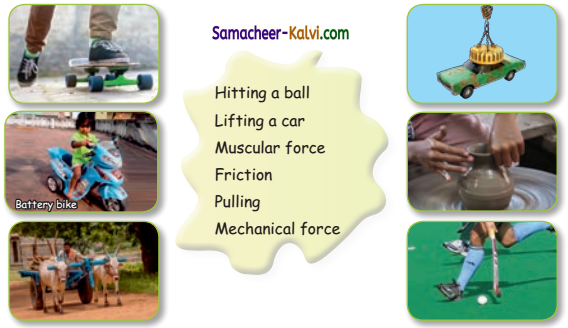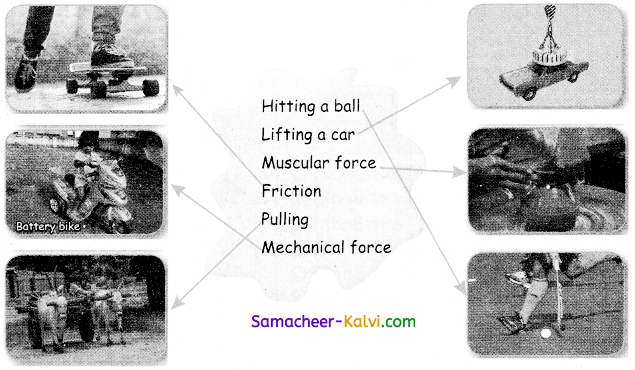Question 1.
How do you open the door?
I open the door by pushing or pulling.

Question 2.
Name the types of force.
i) There are two types of forces.

1. Contact force
2. Non-contact force.

ii) Contact force is classified into three

1. Muscular force
2. Mechanical force
3. Frictional force

iii) Non-Contact force is classified into two.

1. Gravitational force
2. Magnetic force.Question 3.
Which force is involved in collecting water from well?
Muscular force is involved in collecting water from well.

Question 4.
What is push?
When a force is applied in the direction of an object, it is called push.

Question 5.
What kind of force is used to make clay pot?
Muscular force is used to make clay pot.IV. Sujatha places a magnet near some objects. What are the objects that will be attracted from the list given below?
(note, pin, coin, rubber, shirt, comb, steel tumbler, nail)
pin, coin, steel tumbler, nail.

Raja throws a ball, a stone, a paper, and a leaf up in the air? What kind of force is involved here? What will happen to them?
i) Gravitational force is involved here.
ii) So the ball, the stone, the paper, and the leaf will fall down. The earth pulls all the objects towards itself.Intext Activity:

Let us look at the pictures below to understand movement and action better (Text Book Page No. 114):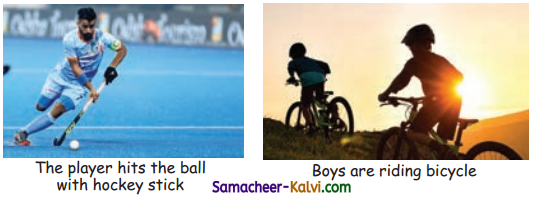In the above pictures.

Question 1.
What moves? _______, ________
The ball, The cycle.

Question 2.
What is the action? _______, ________
Hitting the ball, Riding the bicycle.Put a tick (✓) mark for the pictures of motion (Text Book Page No. 116):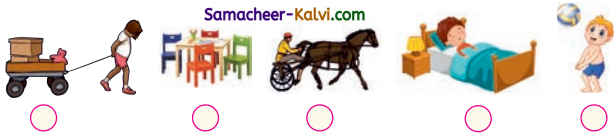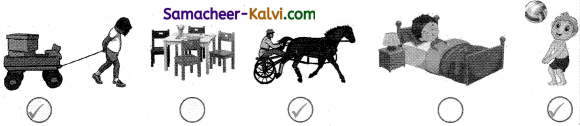Classify the following – Push or Pull (Text Book Page No. 116):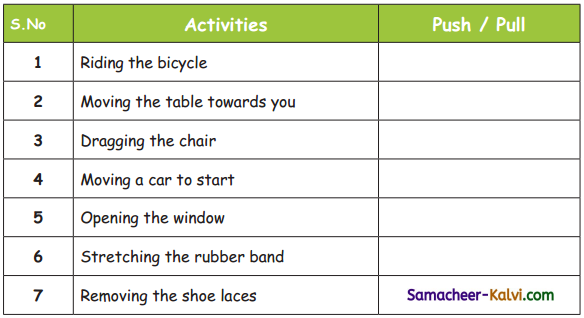S.No Activities Push / Pull 1 Riding the bicycle Push 2 Moving the table towards you Pull 3 Dragging the chair Pull 4 Moving a car to start Push 5 Opening the window Push 6 Stretching the rubber band Pull 7 Removing the shoe laces PullMatch the following (Text Book Page No. 118):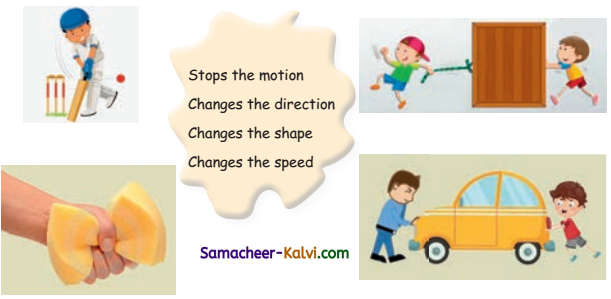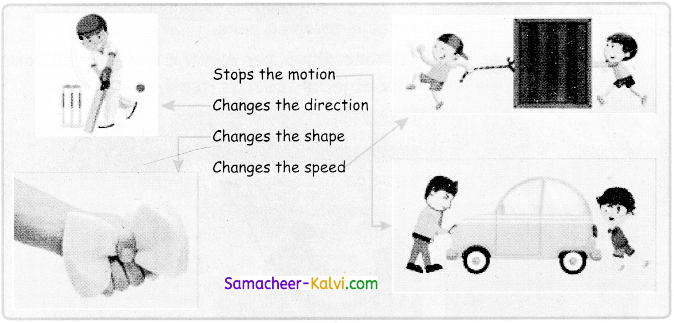Observe the picture and write the kind of force involved here (Text Book Page No. 120):

Question 1.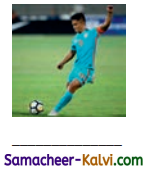Muscular force

Question 2.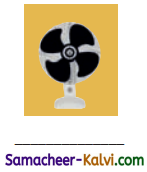Mechanical force

Question 3.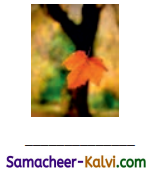Gravitational force

Question 4.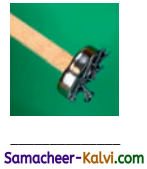Magnetic forceTick (✓) the muscular force (Text Book Page No. 120):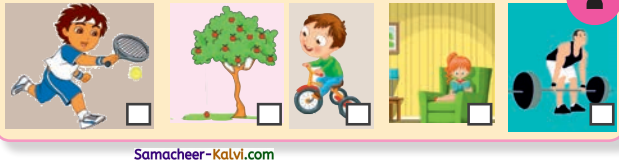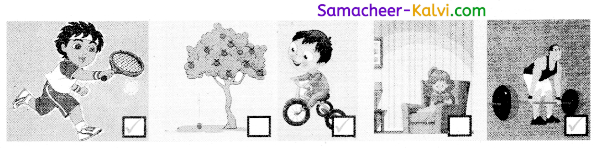Think (Text Book Page No. 121):

Question 1.
Why do we sprinkle the powder on the carrom board before playing carrom?
We sprinkle the powder on the carrom board to make the surface of the board smooth. This reduces the friction between the surface of the carrom board, the striker, and the coins. As a result, the coins and the striker can move easily on the carrom board.

Classify (Text Book Page No. 121):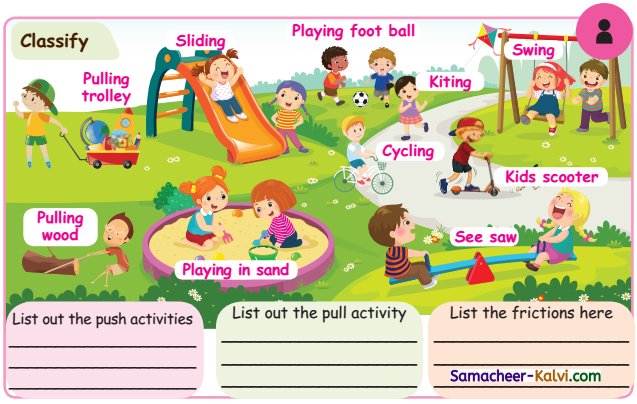List out the push activities List out the pull activity List the frictions here Swing Pulling wood Sliding Playing foot ball Pulling trolley Playing in sand Kids scooter, cycling Kiting See sawQuestion 1.
________ is the motion which gives the required result.
(a) Action
(b) Direction
(c) Shape
(a) Action

Question 2.
________ involves an interaction between two or more objects.
(a) Matter
(b) Force
(c) Action
(b) Force

Question 3.
When a force is applied without touching an object, it is called force.
(a) contact
(b) mechanical
(c) non-contact
(c) non-contactII. Fill in the blanks:

Question 1.
Change in position of an object is called _________.
Ahs :
movement

Question 2.
_________ is required in pulling than in pushing.
Less force

Question 3.
The force applied by using the parts of our body is called _________ force.
muscular

Question 4.
_________ is a force actions between two surfaces in contact.
FrictionIII. Write True or False:

Question 1.
Force can lift or drop an object.
True

Question 2.
The force applied by a machine is called magnetic force.
False

Question 3.
The force between two stones created a spark is called frictional force.
True

Question 4.
Muscular force is a non-contact force.
FalseIV. Answer in a word or two:

Question 1.
Define Motion.